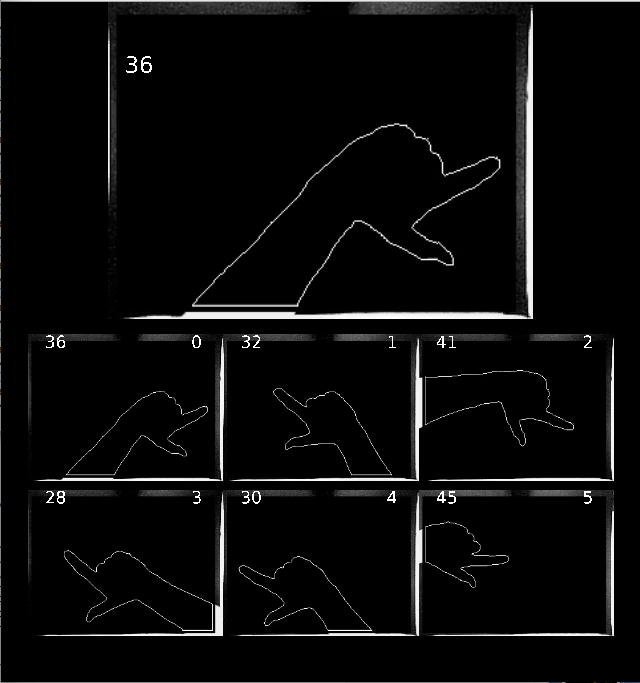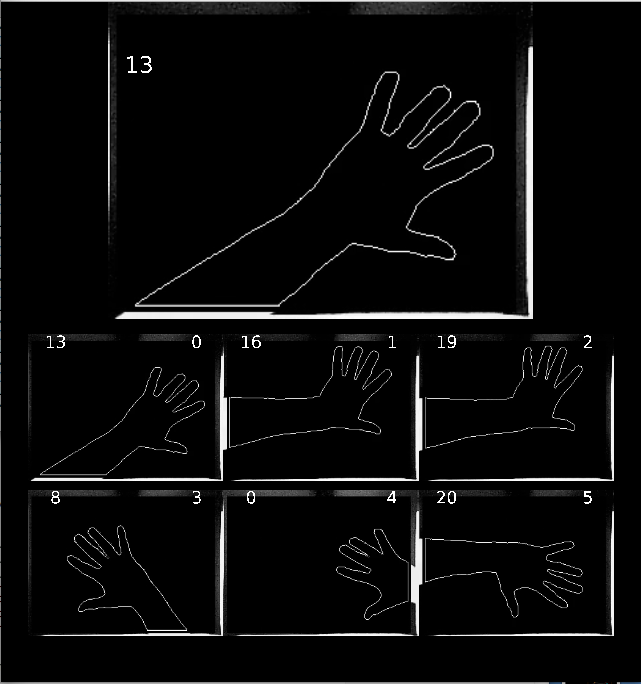# Ircam Residency: Posture Tracking

PART 1: Contour Extraction and Centering.

Two examples

Original Image:

.

.

.

.

.

.

Objective:

.

.

.

.

.

STEP 1)

After low passing the raw contour, find finger Tips and Valleys according to convex and concave local maxima in the angle profile of the contour:

.

.

.

.

.

STEP 2:

Find the bounds of this contour.

.

.

.

.

.

STEP 3:

Add secondary bounds according to a pre-determined target box size in pixels. Place the box away from the point of entry (the side where the contour begins). Fingertips might change the  position of these secondary bounds. The target box size changes with a measure of how “angular “the profile is (if it has a lot of tips and valleys, the angle changes accumulate and give a big “angularity” measure.

.

.

.

.

.

STEP 4:

Choose the bounds that make the closest box:

.

.

.

.

.

STEP 5:

Using the contour inside the box, calculate three points:

1) an entry point (1st and last point / 2),

2) a center based on the average of all points in the contour (green), and

3) a center based on the average of all finger valleys (concave points, yellow)

A weighted center of these three points is obtained, based on two scales:

i. If the angularity measure is high, the entry point has higher weight than the contour average.

ii. the more valleys used for the average, the more we can trust this center.

.

.

.

.

.

STEP 6:

A 1st line is drawn between the entry point and the weighted center, and the contour is cut by a 2nd line perpendicular to the 1st, according to an arbitrary radius around the weighted center.

.

.

.

.

.

STEP 7: A contour is extracted only on the pixels that are on the opposite line of the line cutting at the wrist. A new low pass filter and then angle profile calculation is done.

PART 2: Forward HMM à la gf / rabiner

Each posture has different length angle sequences. There weren’t good results with these sequences so I ended up doing cubic, lagrange interpolation so that each reference observation and each input to the hmm are of length 50.

I used probabilities 0.25 0.50 0.25 for repeating a state, next state, or jumping a state respectively. The algorithm aligns the input to the reference sequences and then finds an error measurement by subtracting them. All of the examples as input are taken from the same vocabulary; i.e. each element in the vocabulary is compared to all of the other elements.

The larger image is the input and the six smaller images are the output. The numbers on the top left are the id of the example, and the numbers on the top right are the order by least error. As you can see, it seems to work…